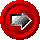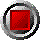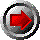Constant Strike Bermudan Swaptions (HW-Tree)Constant Strike Bermudan Swaptions (HW-Tree)

HWTree_BermRecvSwaption_Simple(argument list…)

HWTree_BermPayerSwaption_Simple(argument list…)

These functions return the value per \$1 notional (e.g. 0.03 means 3% of notional) of Bermudan Put (Payer) and Call (Receiver) swaptions, using the Hull-White trinomial tree model. When exercised, the holder of the option enters into a swap with a predetermined start and end date. Accruals begin on the swap start date (if exercised before the swap start date) or on the exercise date (if exercised past the swap start date). These versions of the Bermudan swaption only allow a constant strike rate on evenly-spaced exercise dates (unlike the generalized versions). The functions use the following arguments:

 Argument Description Restrictions Valuation_Date valuation date (e.g. today) valid Excel date number First_Exercise_Date first option exercise date valid Excel date number Exer_TIM the number of months between the first and final exercise dates (0=Euro swaptions) >= 0 (must ensure that it is an even multiple of 12/Exer_Freq) Exer_Freq number of exercise dates per annum 1, 2, 4, or 12 Strike strike rate for the swaption in decimal form (e.g. six percent entered as 0.06) > 0 Notice_Days minimum notice, in days, required to exercise swaption >= 0 Swap_Start_Date beginning of underlying swap valid Excel date number TIM length of underlying swap, in months > 0 Fixed_freq number of fixed-side payments per annum 1, 2, 4, or 12 Yr_Basis year basis used in determining payments 360 or 365 Holidays array of holiday dates valid Excel date numbers strictly ascending order Date_Convention business date convention 0 = no adjustment 1 = Following business day 2 = Modified following 3 = Previous Tree_Steps number of steps in trinomial tree PER EXERCISE DATE > 0, typically 5 to 10 Short_Rate_Vol annual standard deviation of the short rate of interest, in decimal form > 0 Reversion_Rate mean reversion rate of the short rate of interest, in decimal form >= 0 Zero_Dates array of zero coupon curve dates strictly ascending order The first date of this array must be Valuation_Date Zero_Rates array of continuously compounded riskless rates in decimal form (e.g. six percent entered as 0.06) corresponding to Zero_Dates > 0 OAS parallel shift of the zero curve in decimal form Bucket_Start beginning of bucket for zero curve shifts set to 0 if curve shift is not desired Bucket_End end of bucket for zero curve shift >= Bucket_Start Bucket_Shift parallel shift of the zero curve between Bucket_Start and Bucket_End in decimal form set to 0 if curve shock is not desired

© 1995-98 Leap of Faith Research Inc.